# Fiber networks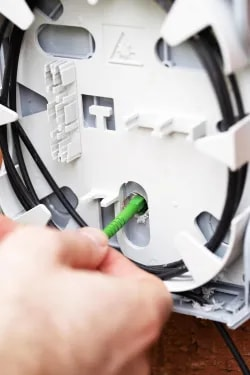Fiber networks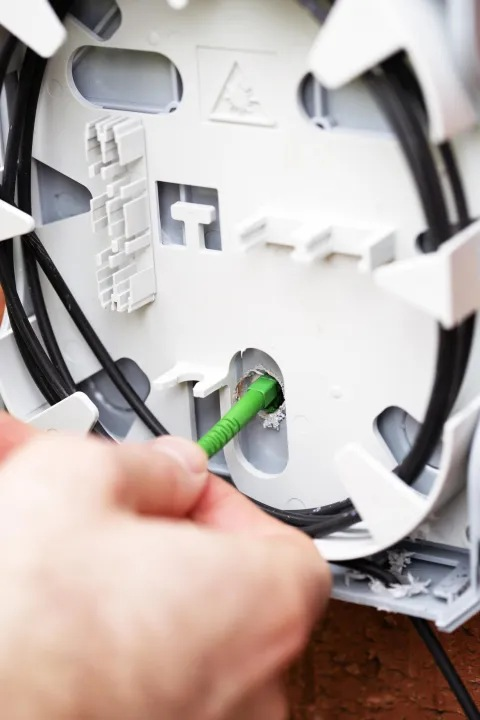Fiber networks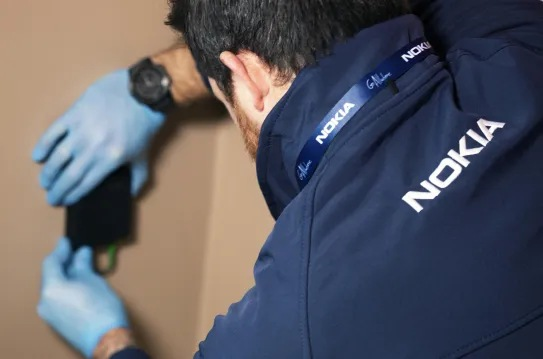Fiber networks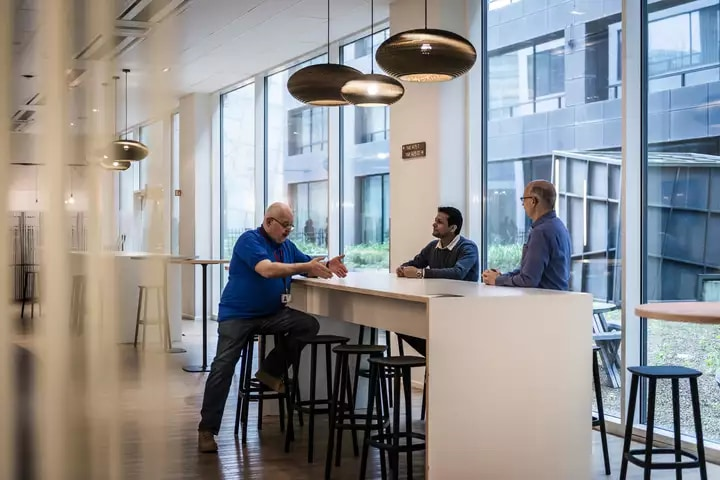Fiber networks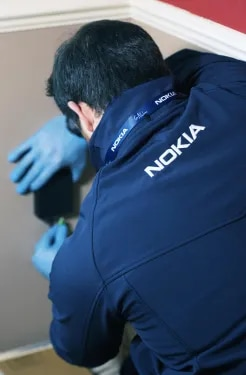Fiber networks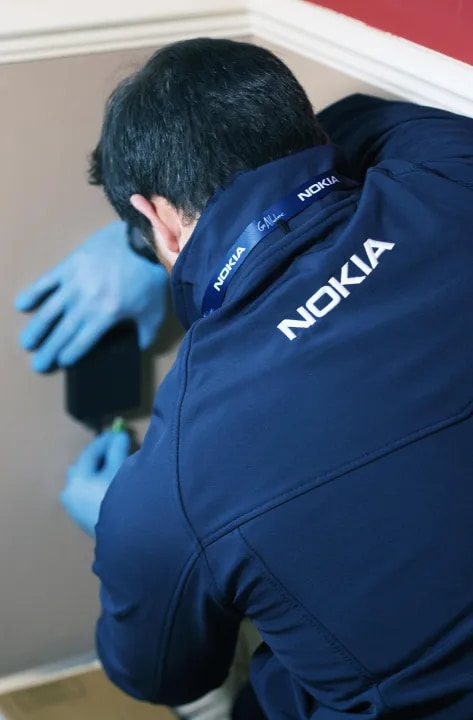Fiber networks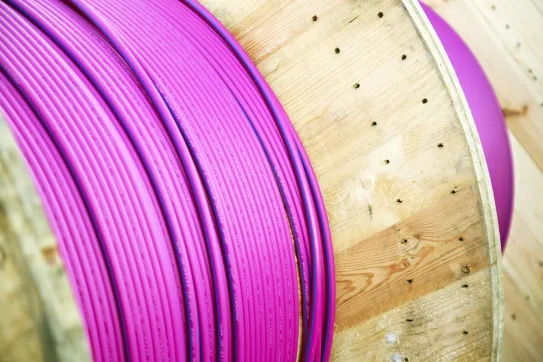Fiber networks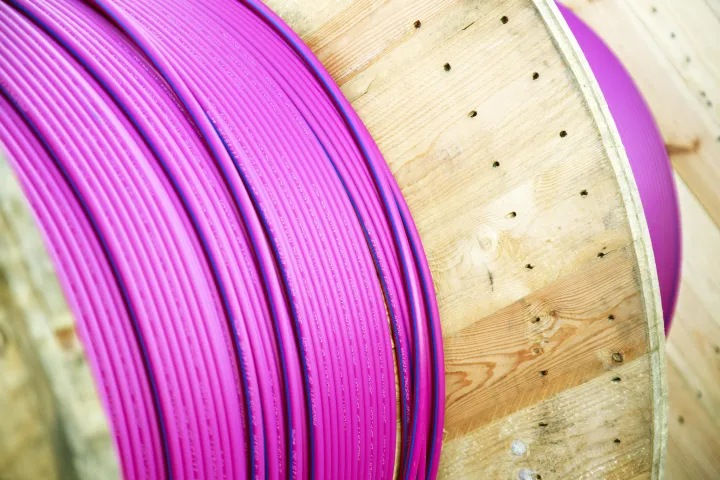Fiber networks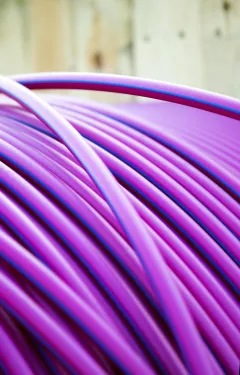Fiber networks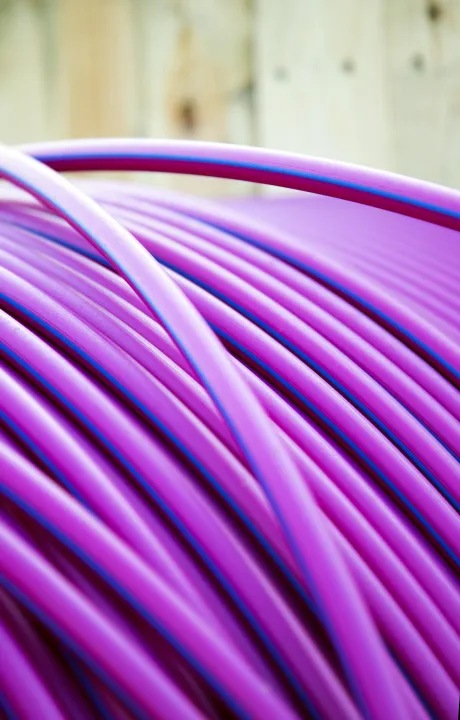Fiber networks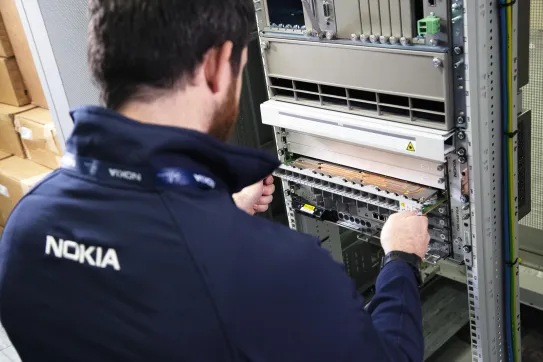Fiber networks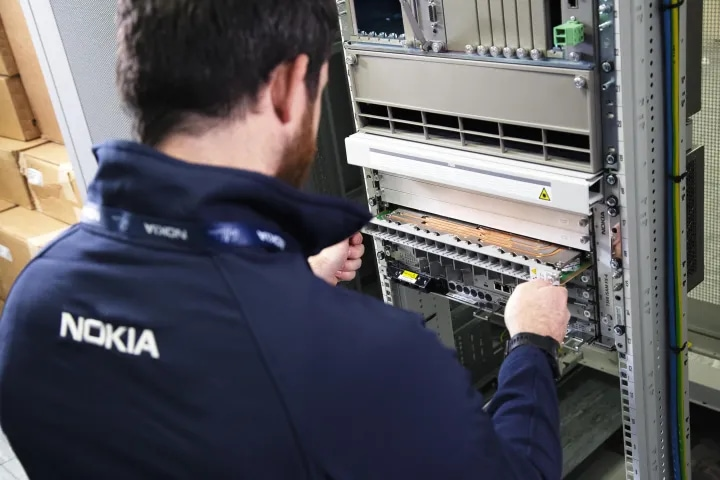Fiber networks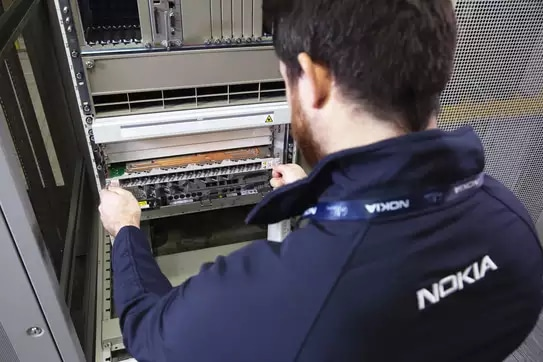Fiber networks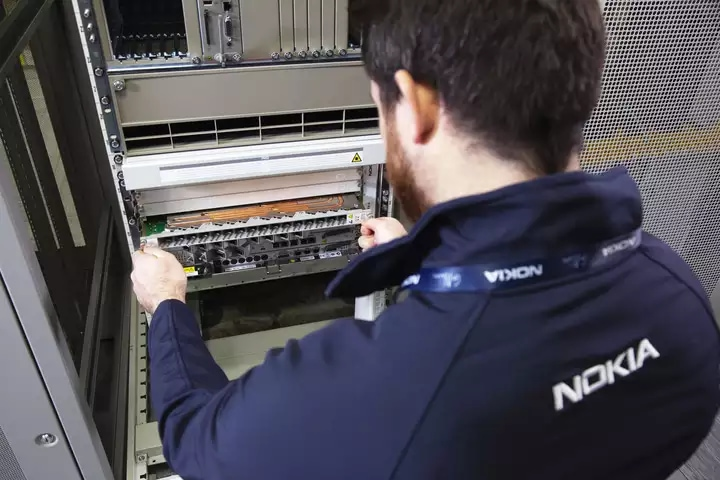Fiber networks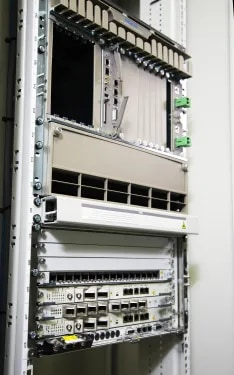Fiber networks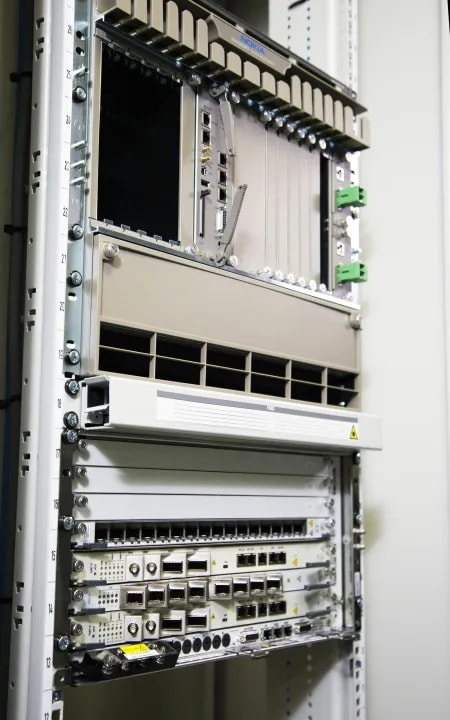Fiber networks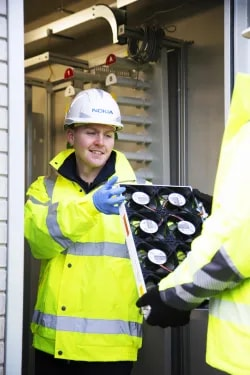Fiber networks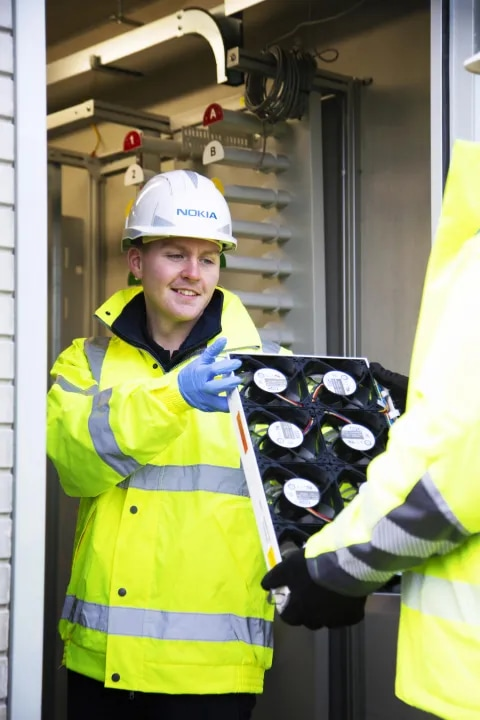Fiber networks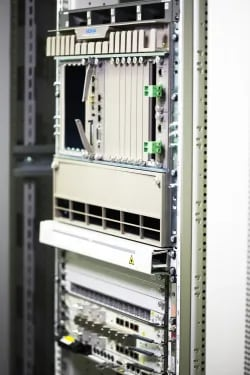Fiber networks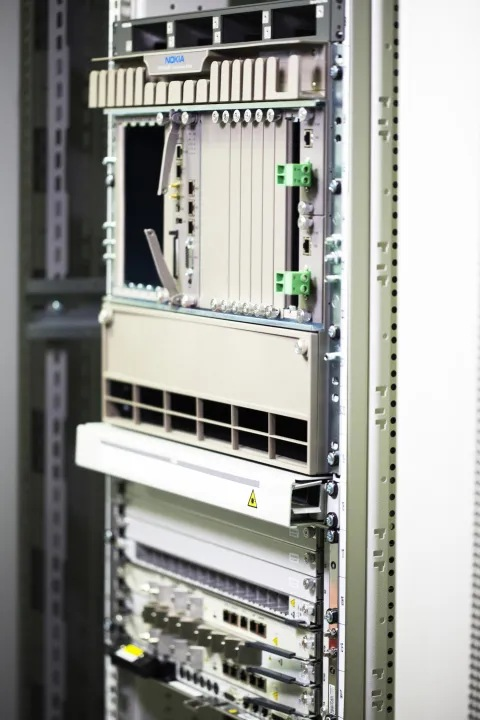Fiber networks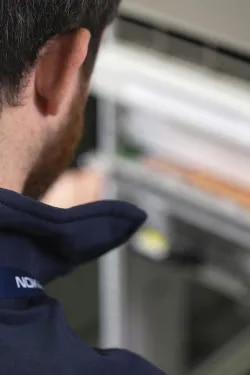Fiber networks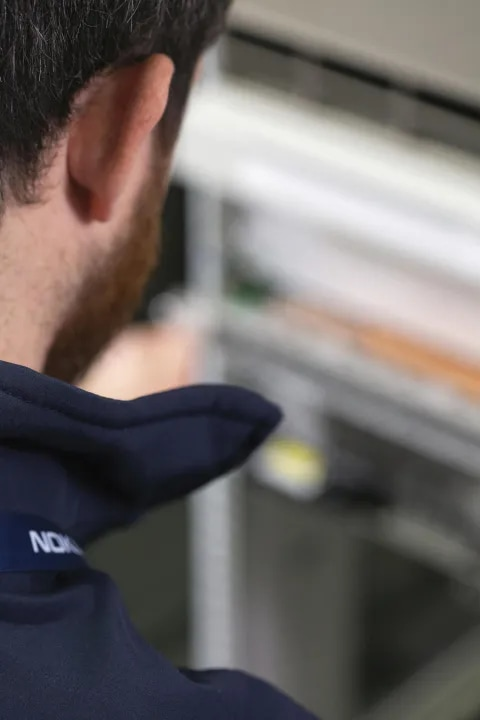Fiber networks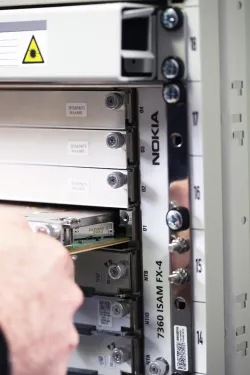Fiber networks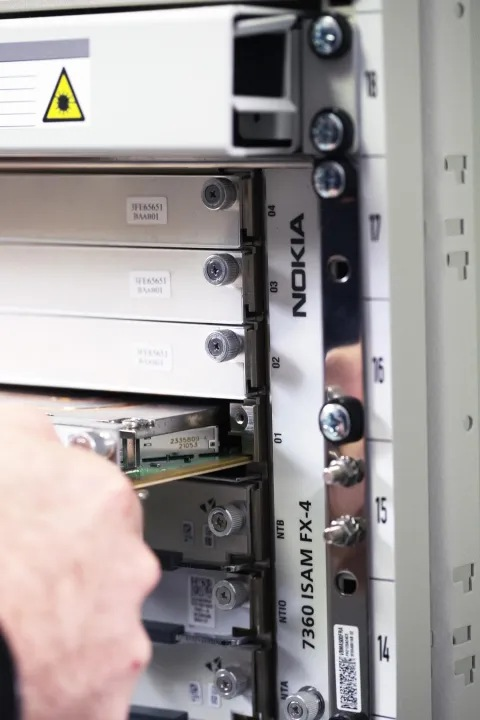Fiber networks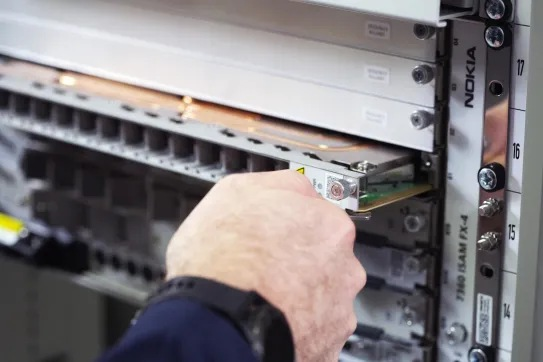Fiber networks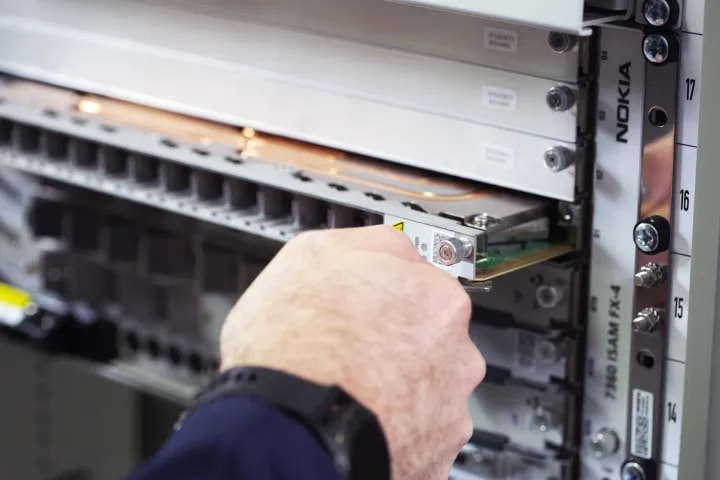Fiber networks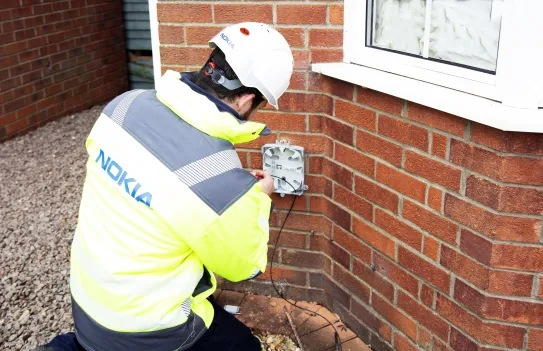Fiber networks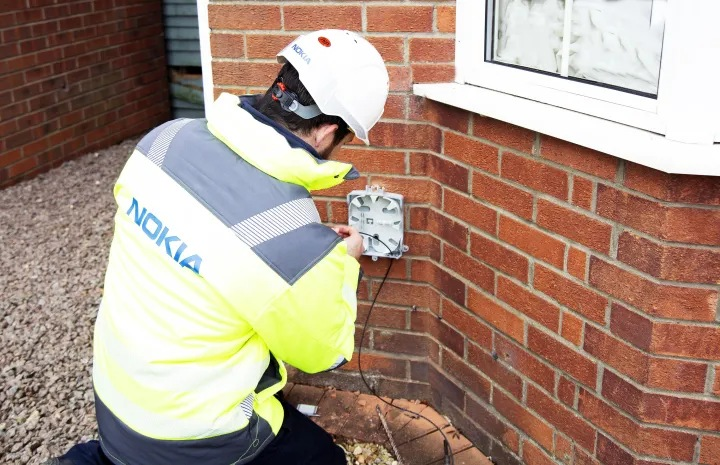Fiber networks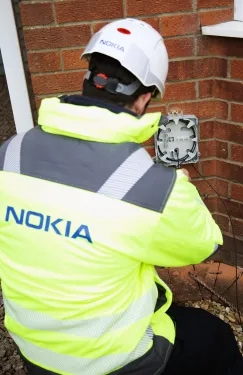Fiber networks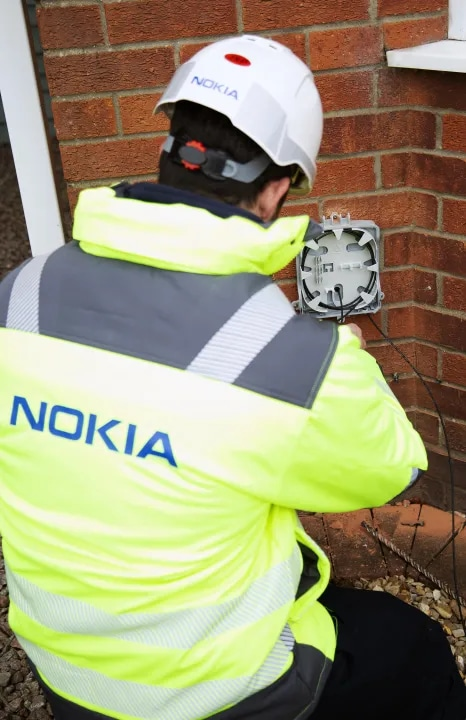Fiber networks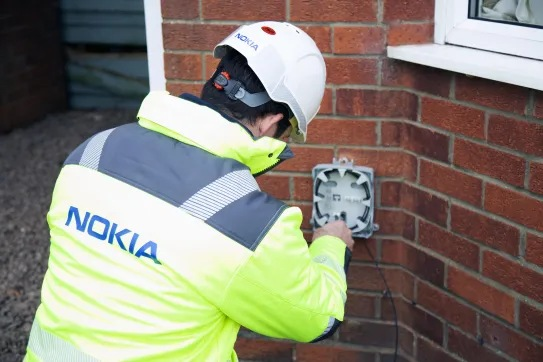Fiber networks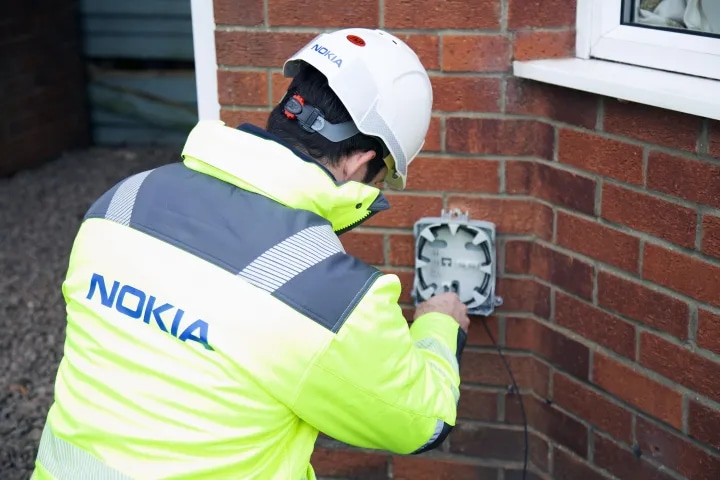Fiber networks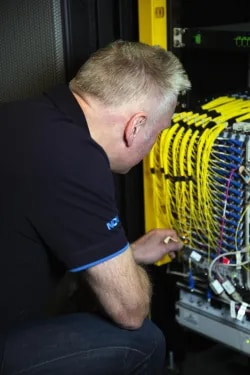Fiber networks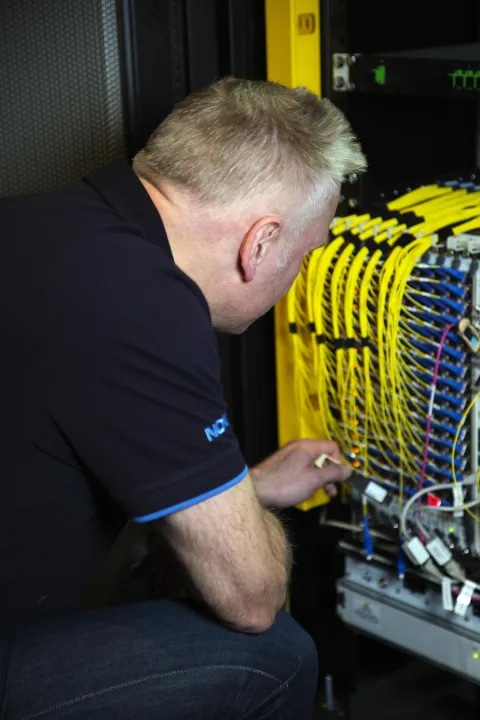Fiber networks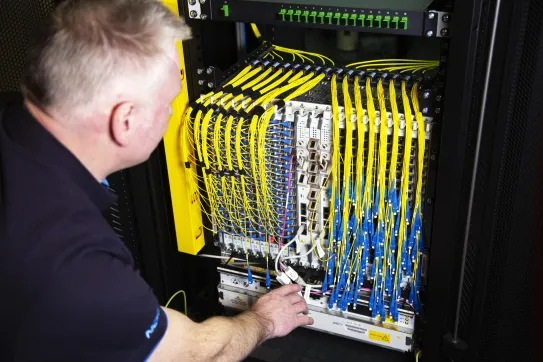Fiber networks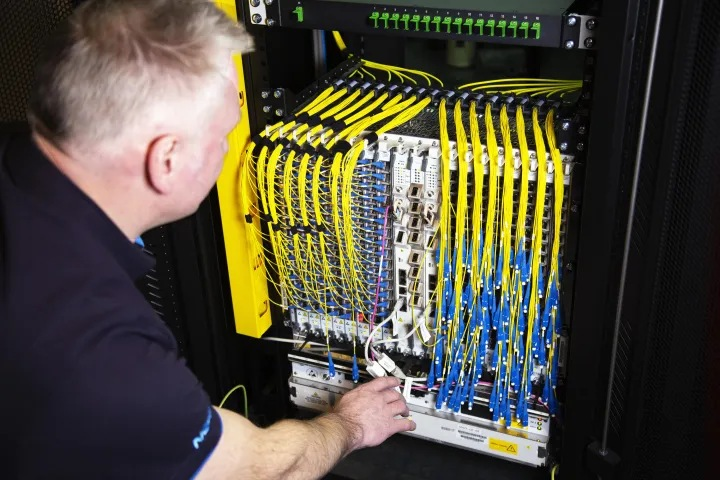Fiber networks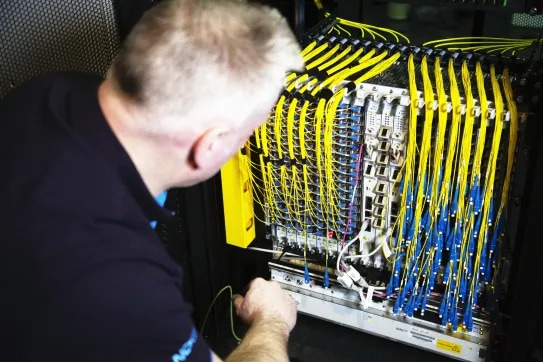Fiber networks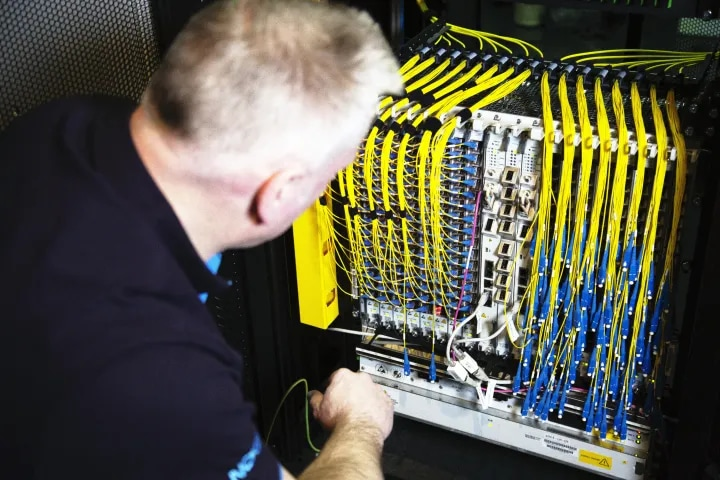Fiber networks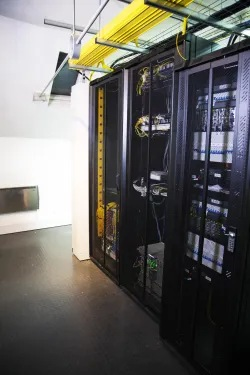Fiber networks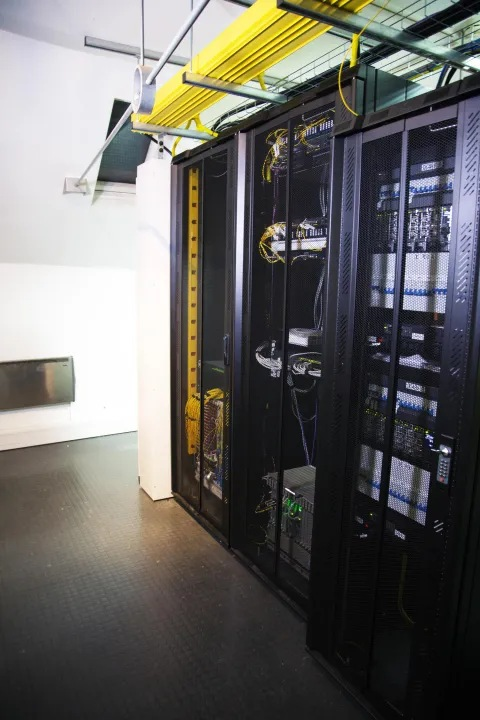Fiber networks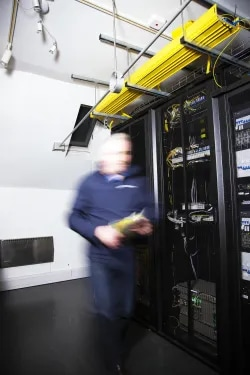Fiber networks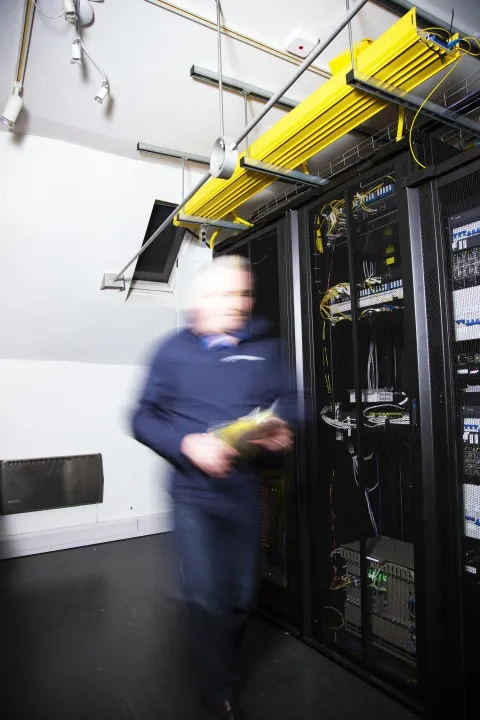Fiber networks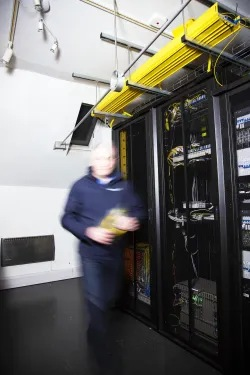Fiber networks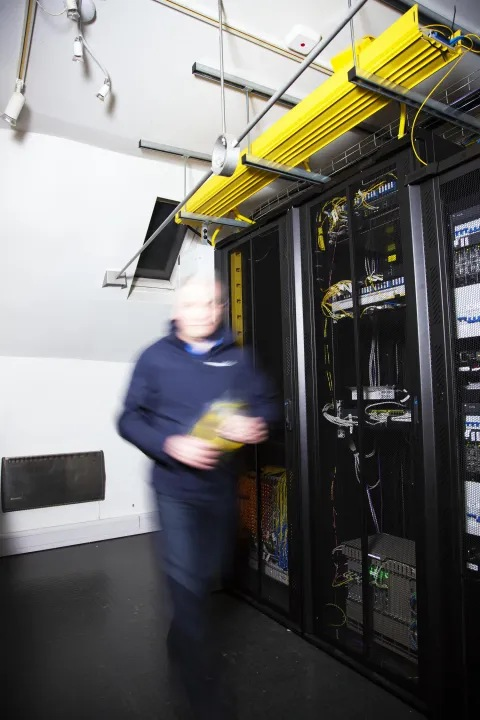Fiber networks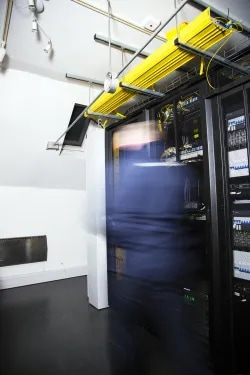Fiber networks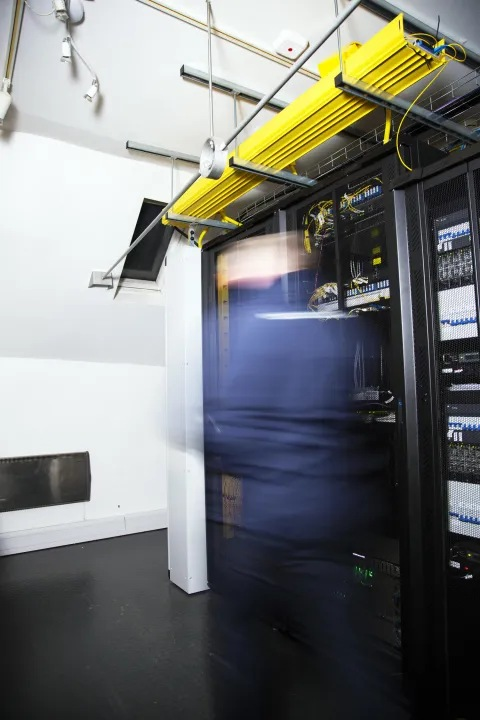Fiber networks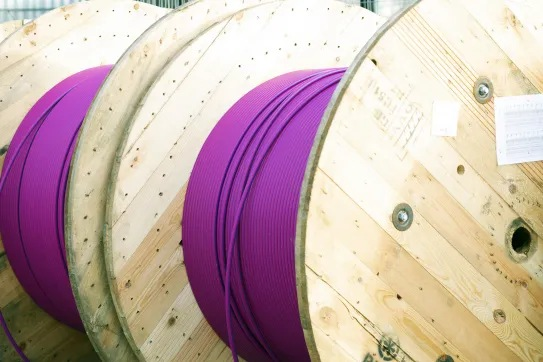Fiber networks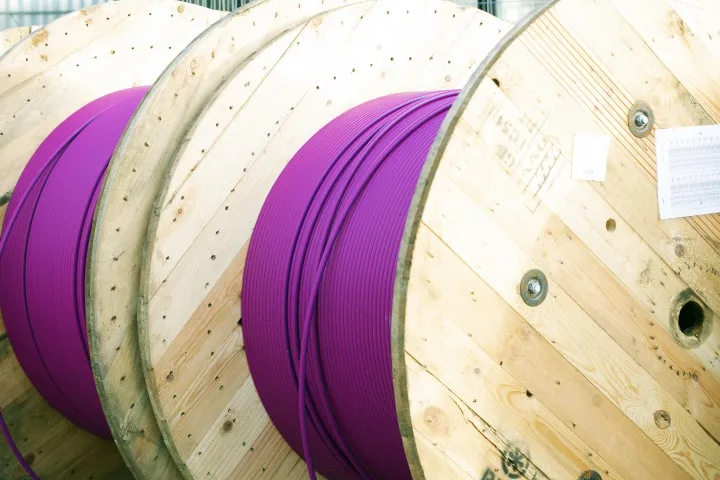Fiber networksFiber networksFiber networks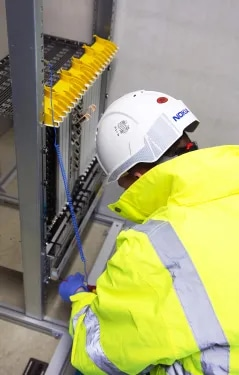Fiber networks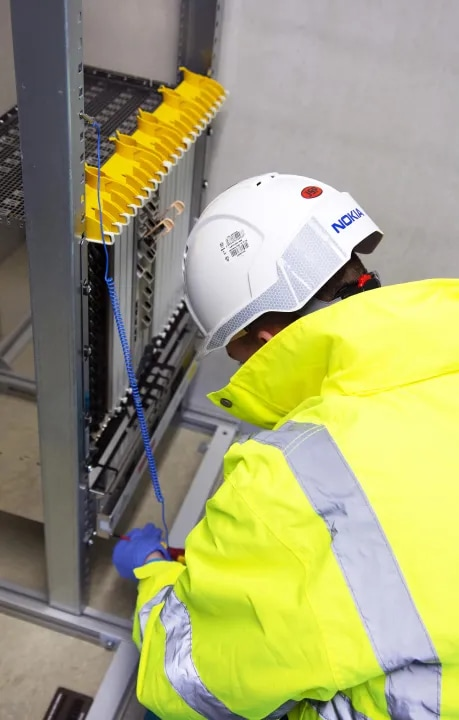Fiber networks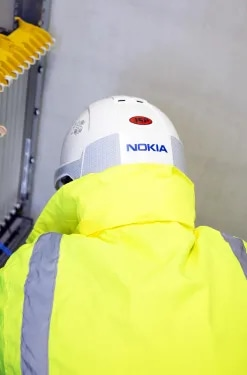Fiber networks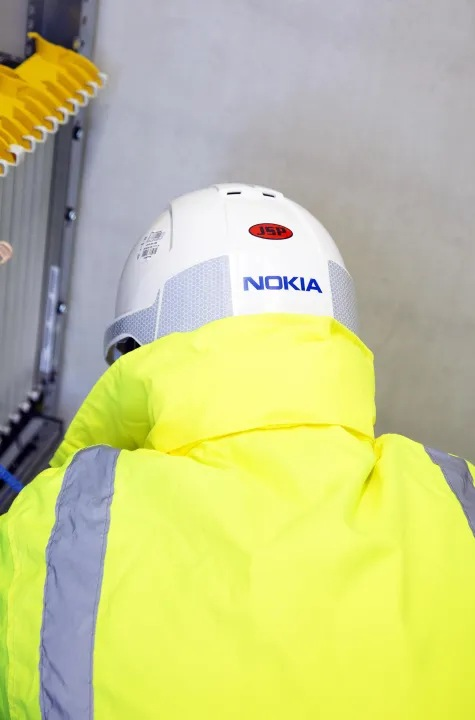Fiber networks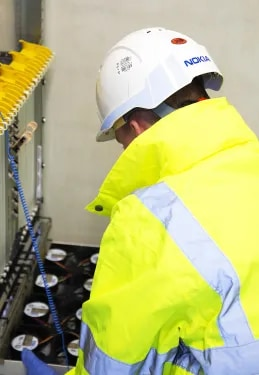Fiber networks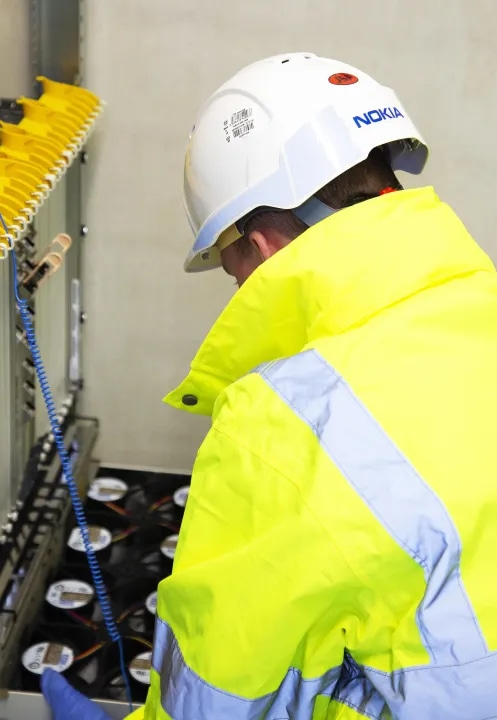Fiber networks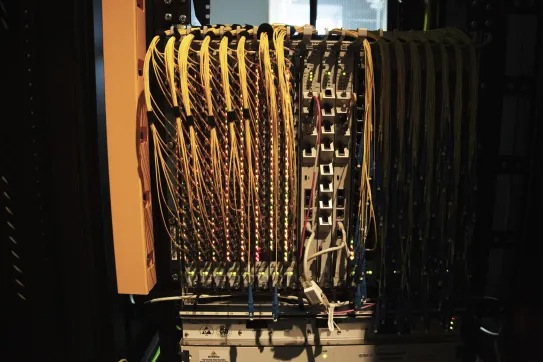Fiber networks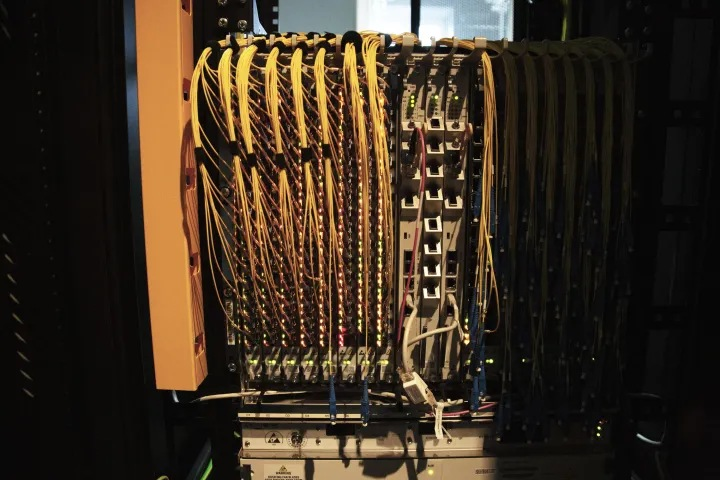Fiber networks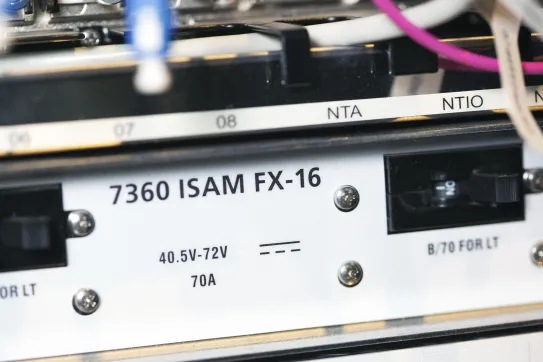Fiber networks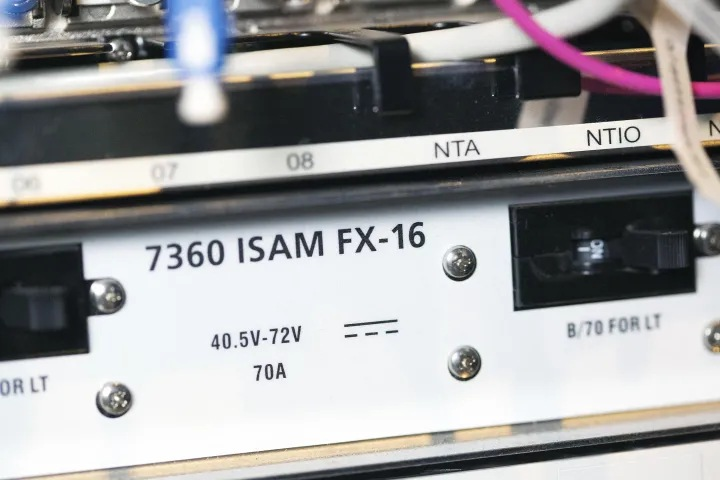Fiber networks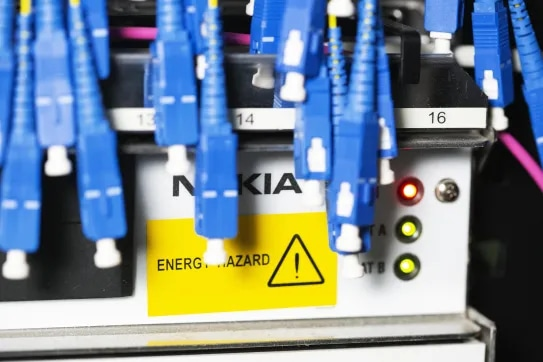Fiber networks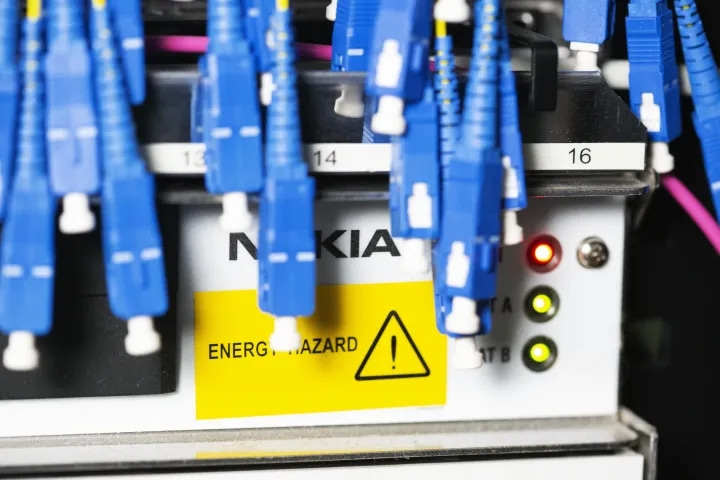Fiber networks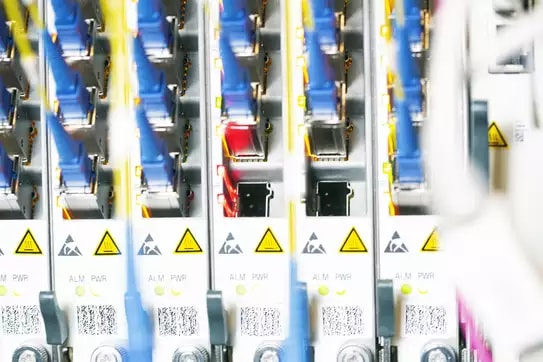Fiber networks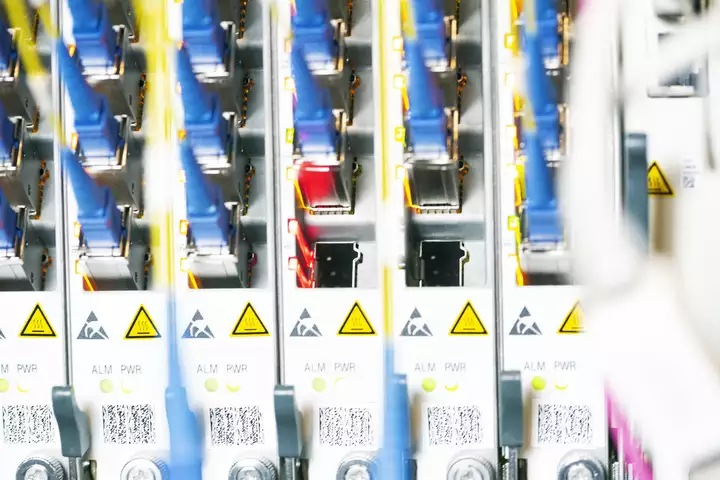Fiber networks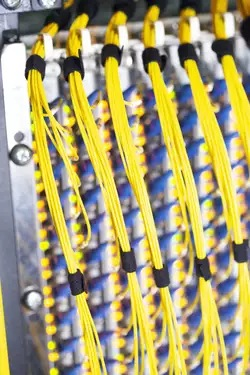Fiber networks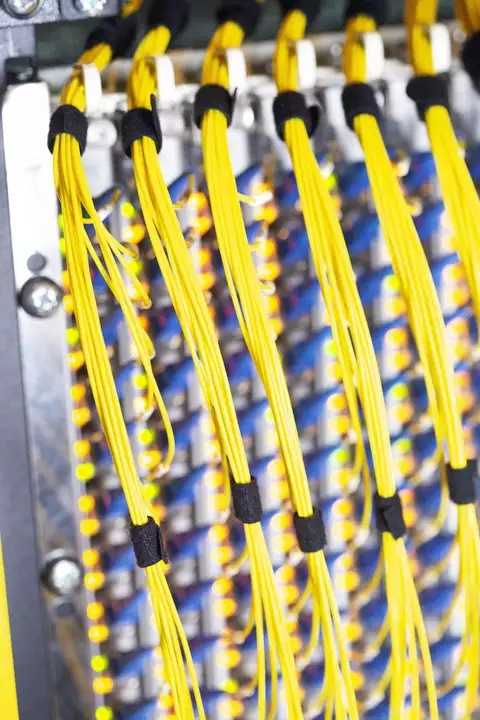Fiber networks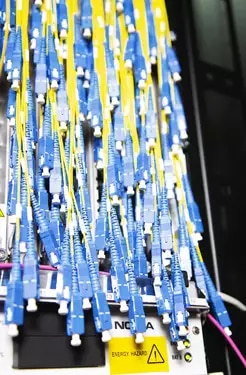Fiber networks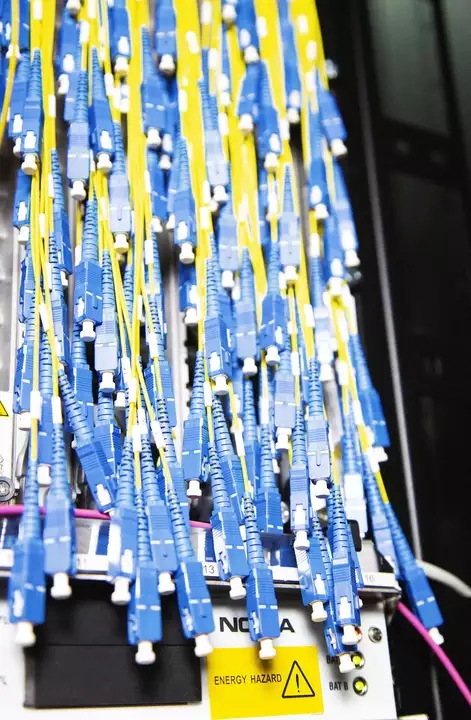Fiber networks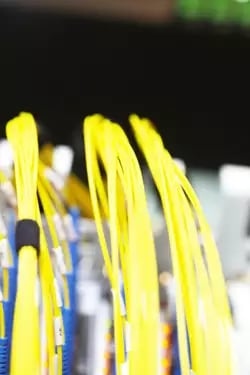Fiber networks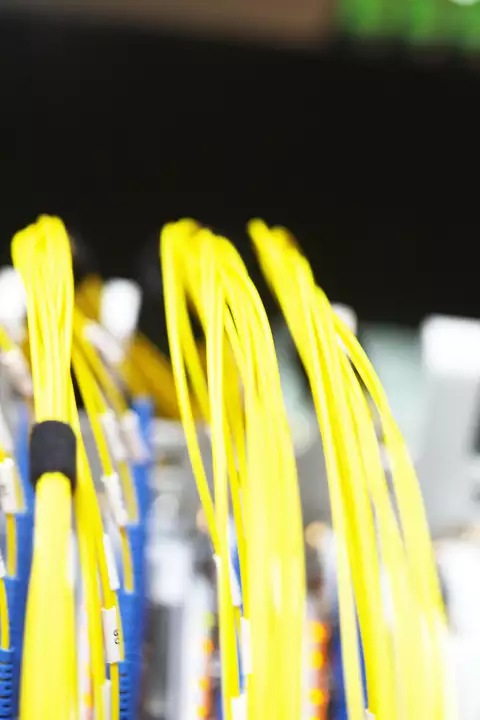Fiber networks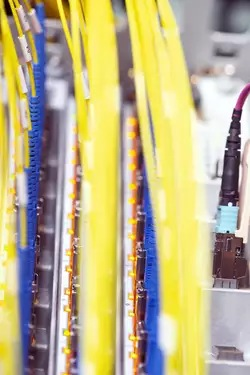Fiber networks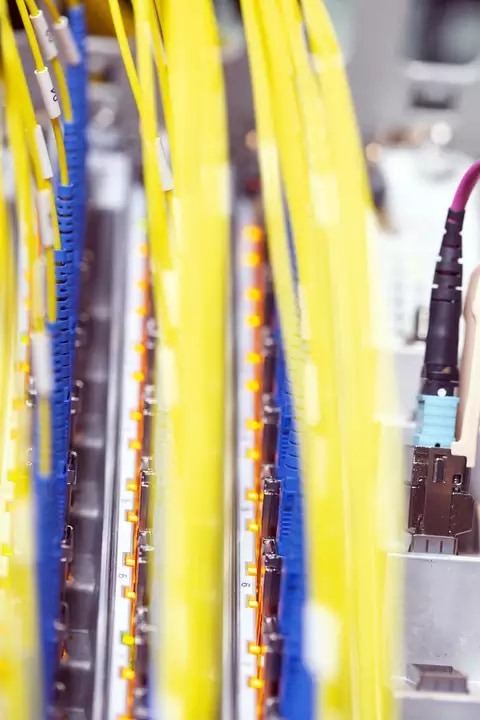Fiber networks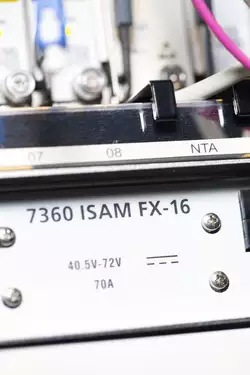Fiber networks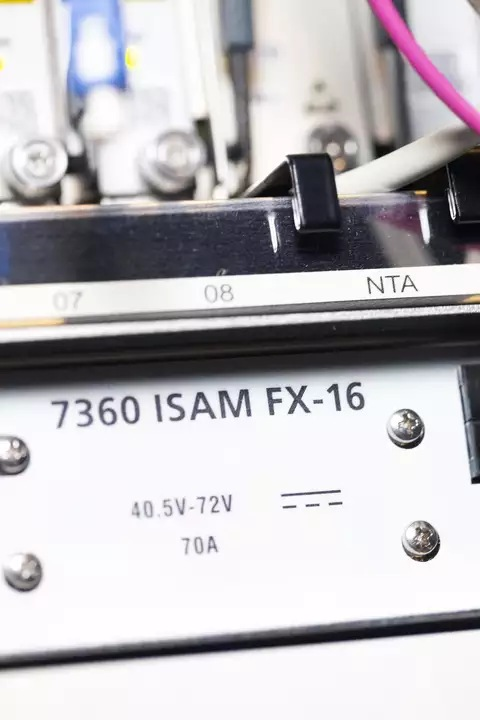Fiber networks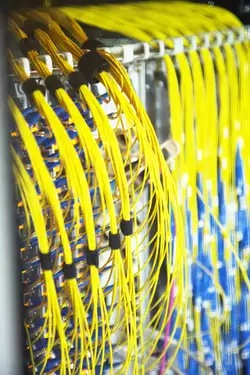Fiber networks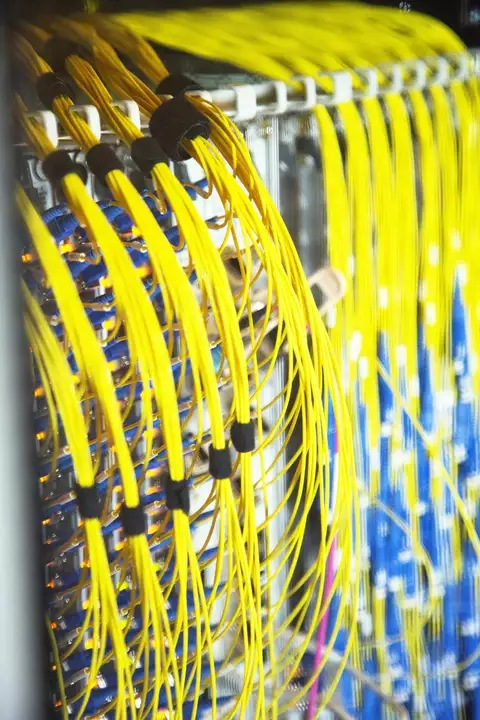Fiber networks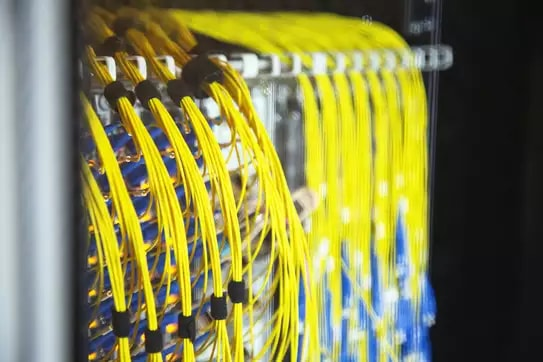Fiber networks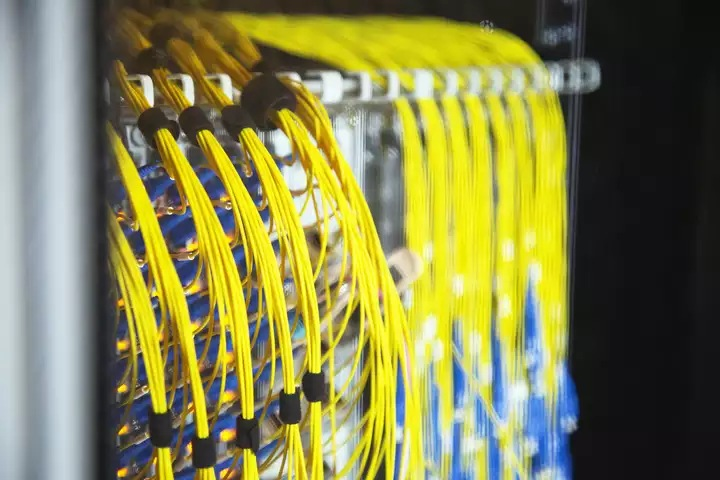Fiber networks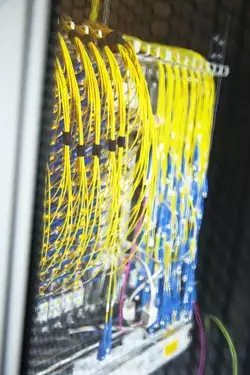Fiber networks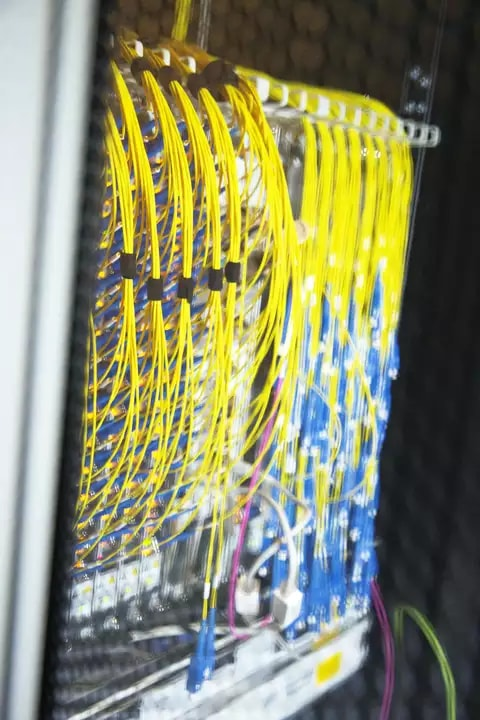Fiber networks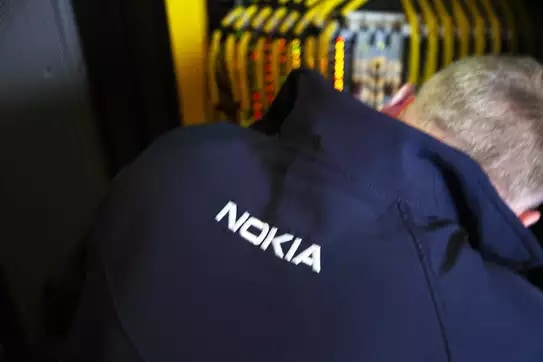Fiber networks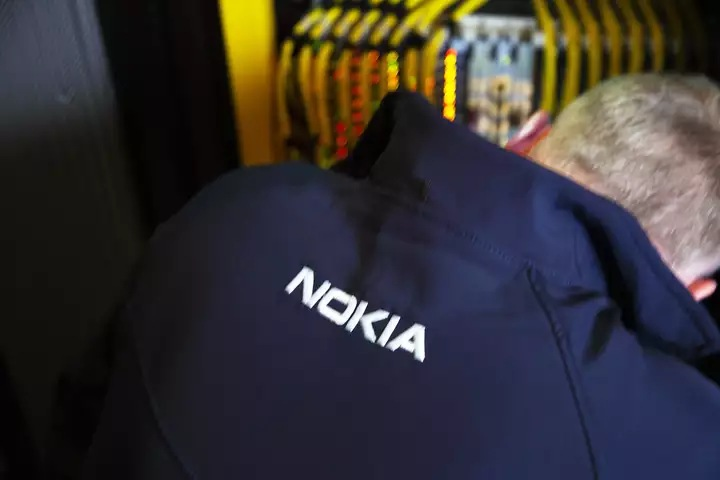Fiber networks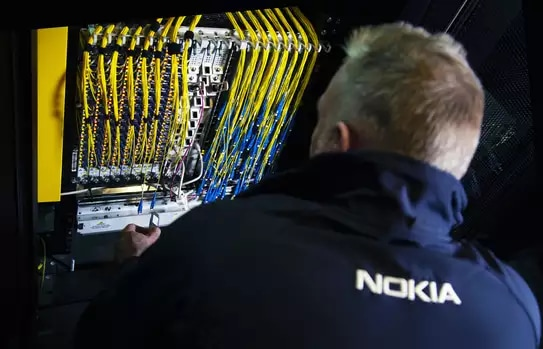Fiber networks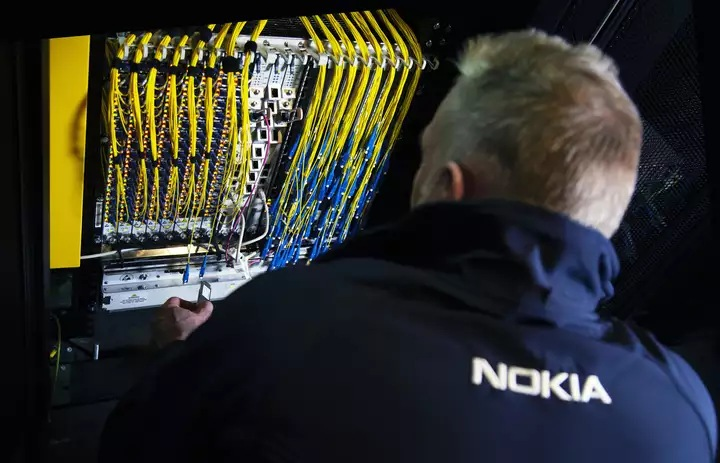Fiber networks